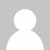A couple of days ago I mentioned that my utils library is slowly growing with LINQ-like methods as I’ve worked through solving the daily Advent of Code challenges this year. For today’s puzzle, I needed to create a new `zip` method, as well as use `count` and `take`.

The puzzle required us to generate a sequence of numbers, and to do so I created an ES6 generator function capable of generating the sequences we needed for both parts of the puzzle:

``````function *generator(seed,factor,test) {
let cur = seed;
for(;;) {
cur = (cur*factor)%2147483647
if (!test || test(cur)) yield cur;
}
}
``````

This method generates an unending sequence, but we only needed to take a certain number of elements from the sequence (40 million for part 1, 5 million for part 2). So I need a `take` method that takes the first `n` elements from an iterable sequence. Here’s my implementation:

``````function *take(seq, count) {
let n = 0;
for(let el of seq) {
if (++n > count) return;
yield el;
}
}
``````

Next, we were comparing elements in two sequences, and that’s where a `zip` method comes in handy. My `zip` method iterates through both sequences, yielding a two element array containing the pair of elements at the same position. It exits when either sequence ends.

``````function *zip(seq1,seq2) {
let it1 = seq1[Symbol.iterator]()
let it2 = seq2[Symbol.iterator]()
for(;;) {
let el1 = it1.next()
if(el1.done) return;
let el2 = it2.next()
if(el2.done) return;
yield [el1.value,el2.value]
}
}
``````

And finally we needed to count how many elements in the zipped sequence matched a certain condition. I could do this by filtering my sequence with my `where` utility method and then passing it into my `count` method. But I decided to upgrade my `count` method to take an optional predicate to count all elements that match a certain condition:

``````function count(seq, predicate) {
let n = 0;
for(let el of seq) {
if (!predicate || predicate(el)) n++
}
return n;
}
``````

With these very generic and reusable utility methods at my disposal, solving the challenge becomes very easy. I can combine them to count all the elements in the pair of sequences that match according to a specific rule:

``````function countMatch(seq1,seq2,n) {
const comp = ([a,b]) => (a&0xFFFF) === (b&0xFFFF); // brackets are needed!
return count(take(zip(seq1,seq2),n),comp)
}
``````

One gotcha I ran into was the operator precedence of my comparison. The equality comparison clearly has higher precedence than the bitwise AND operator which was not what I was expecting:All that remained was to parse my input and then use my `generator` and `countMatch` functions to solve both parts of today’s puzzle:

``````function solve(input, part) {
const parse = s => Number(/\d+/.exec(s))
let genAStart = parse(input)
let genBStart = parse(input)
const aFactor = 16807, bFactor = 48271

if (part === 1)
return countMatch(generator(genAStart,aFactor),
generator(genBStart,bFactor), 40000000);
else
return countMatch(generator(genAStart,aFactor,n=>(n%4)===0),
generator(genBStart,bFactor,n=>(n%8)===0), 5000000);
}
``````

As my utils library is slowly growing, I’ve started to break out the Jasmine tests for each utility out into their own “Spec”. It’s important to keep this utility code well covered by tests as these methods are being relied on in more and more places, and are slowly picking up new capabilities, such as the optional predicate for the `count` function. A good suite of unit tests protects against regressions and also is invaluable when attempting performance optimizations. Maybe I’ll see if I can turn my utils library into an npm package once I’m done.